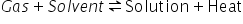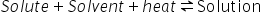# Types of Solutions

SOLUTIONS

A solution is a homogeneous mixture to two or more chemically non reacting substance whose composition can be varied with in certain limits. Depending upon the number of components the solution may be binary (two components), ternary (three components) etc. but in the present unit we will be discussing the binary solutions i.e. the solution(S) containing two components-solute(A) and solvent(B). solute is the component which is present in small amounts and solvent is the component present in more amounts. Depending upon the nature of solute and solvent is the component solutions are of nine types. Out of these a few important types are discussed as below:-

1. Solution of Gases in Liquids :- the solution in which Gas is solute and liquid is solvent is called solution of gas in liquid. Gases dissolve in liquids in liquids to form homogeneous mixture. The solubility of gas in a liquid depends upon the following factors :-
1. Nature of the gas in liquid:- gases like hydrogen, oxygen, nitrogen etc dissolve in water to a small extent where as gases like CO2, HCl, NH3 are highly soluble. Also the gases oxygen and nitrogen are more soluble in ethyl alcohol then in water, while H2S, NH3 etc are more soluble in water than in alcohol. The greater solubility of the gas in liquid is due to the chemical similarity between the gas and solvent.
2. Effect of Temperature:- the solubility of a gas decreases with increase in temperature. The dissolution of gas in a liquid is an exothermic process i.e.Hence on increasing temperature the equilibrium will shift in backward direction according to Le-Chatlier principle i.e. the solubility decreases.

3. Effect of pressure:- the effect of pressure on the solubility of a gas in liquid is explained with the help of Henry’s Law.

This law states that – “The mass of a gas dissolved per unit volume of the solvent at a given temperature is proportional to the pressure of the gas in equilibrium with the solution.” i.e.

M α p                    m = mass of gas,  p = pressure of gas

Or m = k x p        where k = constant

The Henry’s Law may also be stated in terms of mole fraction of gas as – “The pressure of a gas over a solution in which the gas is dissolved is proportional to the mole fraction of the gas dissolved in the solution”

i.e. p α xA             xA = M.F. of gas of solution

or  p= KH X xA where  KH = Henry’s Law constant

The value of KH must be taken in (Atm or bar)

Application of Henry’s Law:-

1. CO2 is used as a preservative in soft drinks hence to increase the solubility of CO2 in drinks; they are sealed under high pressure.
2. In deep sea diving a mixture of O2, He and N2 is used for breathing. It prevents the effect of decrease in pressure.
3.  The transport of oxygen by hemoglobin to other parts of body is carried out by Henry’s Law.

Limitations of Henry’s Law :-

1. The pressure should be low and temp. should be high i.e. the gas should behave ideally.
2. The gas should not under go compound formation with the solvent or associate or dissociate in solvent. e.g. Henry Law is not applicable to NH3 and HCl in water.

(2) Solution of solid in Solid (solid solutions):- Solid solutions are those solutions in which both the components i.e. solute and solvent are solids. These are classified into two types.

• Substitutional solid solutions:- if some atoms, ions or molecules of one solid present at the lattice sites are replaced (substituted) by the atoms, ions or molecules of another solid having similar sizes, the solids thus obtained are called subsitutional solids (fig.) e.g. Brass, Bronze, steel etc.

Interstitial Solids Solution:- In the lattice of a solid (host), if the atoms of some other solid, small in size, occupy the interstitial sites or voids, the solid obtained is called interstitial solid lattice and Carbon atoms occupies the octahedral voids.(3) Solution of solids in liquids:- the solution in which solid is solute and liquid is solvent is called solution of solid in liquid.

Factors affecting the soluting of a solid in a liquid:- the important factors on which the solubility of solid in liquids depends are:

1. Nature of solute and solvent:- in general a solid dissolves in a liquid which is chemically similar to it. This is expressed by the maxim “like dissolves like”. It means that polar (ionic) compounds dissolve in polar solvents like water, while non polar compounds dissolve in non polar solvents like benzene, ether, carbon tetrachloride etc.
2. Effect of temperature on solubility (solubility curves):- On the basis of the effect of temperature on solubility the various substance are divided into three parts:
1. Whose solubility increases continuously with increase of temperature:- the substances for which the process of dissolution is endothermic fall under this category e.g.Applying Le-Chatlier’s principle if temp. is increased, equilibrium will shift in that direction in which heat is absorbed i.e. in the forward direction and dissolution in more. For example

NaNO3, KNO3, NaCl, KCl etc.

2. Whose solubility decreases continuously with increase of temperature:- These are the substances for which the process of dissolution is exothermic e.g. cerium sulphate, Li2CO3, Na2CO3 etc.
3. Whose solubility does not increase or decrease continuously:- it occurs in case of the compounds which on heating changes from one polymorphic form of another. The temperature at which a state changes into another is called transition temperature.

The plot of solubility against temperature is called the solubility curves. There is a continuous curve of types (a) & (b) and discontinuous curve for type (c).

Related Keywords